• 主要介绍了C++通过自定义函数求一元二次方程,涉及C++数学运算相关技巧,非常简单实用,需要的朋友可以参考下
• 主要介绍了Python编程实现数学运算求一元二次方程的实算法,涉及Python数学运算求解方程的相关实现技巧,需要的朋友可以参考下
• 通过java实现输入系数求一元二次方程根，学习Scanner()、split()和Substring()函数-附件资源
• ## Java求一元二次方程的根

千次阅读 多人点赞 2018-10-29 22:45:01
求一元二次方程。 【问题描述】编写程序，从键盘输入三个系数ax2+bx+c=0，计算方程的解并输出。需要考虑方程有虚根、方程有实、方程是一元一次方程、没有等。输出方程的解并保留6位小数。 import java....
求一元二次方程的根。
【问题描述】编写程序，从键盘输入三个系数ax2+bx+c=0，计算方程的解并输出。需要考虑方程有虚根、方程有实根、方程是一元一次方程、没有根等。输出方程的解并保留6位小数。
import java.text.DecimalFormat;
import java.util.*;
public class  Equation{
public static void main(String[] args) {
System.out.println("请输入方程的系数a、b、c：");
int a, b, c;
DecimalFormat df = new DecimalFormat("0.000000");
int delta;
double x1, x2;
delta = b * b - 4 * a * c;
if (a == 0 && b == 0 && c == 0) {
System.out.println("方程有无限根。");
return;
}
if (a == 0 && b == 0 & c != 0) {
System.out.println("方程无解。");
return;
}
if (delta == 0) {
x1 = b / -2.0 / a;
x2 = b / -2.0 / a;
System.out.println("方程有两个相同实根, x1 =  "
+ df.format(x1) + ",  x2 = " + df.format(x2));
}
if (delta > 0) {
x1 = (-b + Math.sqrt(delta)) / 2.0 / a;
x2 = (-b - Math.sqrt(delta)) / 2.0 / a;
System.out.println("方程有两个不同实根, x1 = "
+ df.format(x1) + ",  x2 = " + df.format(x2));
}
if (delta < 0) {
double s, x;
s = -b / 2.0 / a;
x = Math.sqrt(-delta) / 2.0 / a;
System.out.println("方程有虚根, x1 = "
+ df.format(s) + " + " + df.format(x) + "i,  x2 = "
+ df.format(s) + " - " + df.format(x) + "i");
}
}
}

运行结果：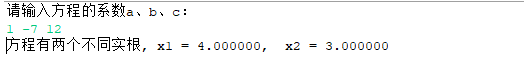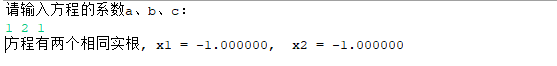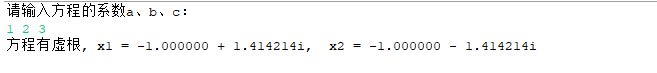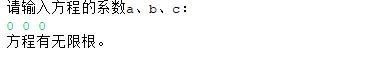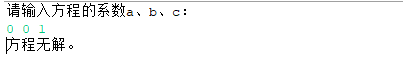展开全文Java
• 通过java实现输入系数求一元二次方程根，学习Scanner()、split()和Substring()函数 学习目的： 1、针对scan未关闭问题处理 2、参数输入，可以用scan直接获取，也可以用split()分割 3、Substring()获取子字符串...
通过java实现输入系数求一元二次方程根，学习Scanner()、split()和Substring()函数
学习目的：  1、针对scan未关闭问题处理  2、参数输入，可以用scan直接获取，也可以用split()分割  3、Substring()获取子字符串学习
1、用try{}catch{} finally{}解决函数未关闭问题
问题提示，如下（有时未必会影响最后输出结果）：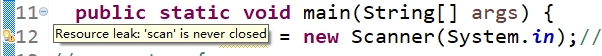解决代码：
  try {  ......    //省略操作代码
}
catch(Exception e){     //输出错误提示信息
System.out.println(e);
}
finally {   //不管什么情况都会执行，都会执行关闭scan
scan.close();
}
加入try{}catch{} finally{} 后，提示显示，当然一些程序也可以直接在末尾加入scan.close()语句关闭，根据代码需要判断！
2、二次方程参数获取
（1）直接用Scanner（）输入获取，然后用Double.parseDouble()，将获取参数转换为double型：
        System.out.println("请输入:a、b、c:");//输出提示信息
String a1=scan.next();  //获取第一个输入元素并赋值给a1
String b1=scan.next();  //获取第一个输入元素并赋值给b1
String c1=scan.next();  //获取第一个输入元素并赋值给c1
（2）用正则表达式，并用split()分割字符串
   System.out.println("请输入:a=?&b=?&c=?");//输出提示信息
String str = scan.next();//记录键盘录入的字符串
String regex = "[&]";//正则表达式
String[] arr = str.split(regex);//按正则切开
for(int i=0;i<arr.length;i++) {//遍历输出处理后的字符串数组
System.out.println(arr[i]);
}
该部分输出如下：a=1 b=6 c=4，同样用Double.parseDouble()，将获取参数转换为double型：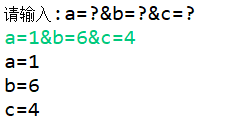3、Substring()函数获取子字符串
（1）接下来我们要提取输出的1、6、4元素，由上面输出我们知道有arr元素为a=1,arr元素为b=6,arr元素为c=4,我们由以下可实现得到a1=1,b1=6,c1=4.
      String a1 = arr.substring(2);//取出字符串数组中第一个元素从"="开始到结尾的字符串，substring(int beginIndex)
String b1 = arr.substring(2);//取出字符串数组中第二个元素从"="开始到结尾的字符串
String c1 = arr.substring(2);//取出字符串数组中第三个元素从"="开始到结尾的字符串
（2）substring()函数简介  substring()方法可以对字符串进行截取。
1> substring(int beginIndex)

该方法返回从指定的索引位置开始截取直到该字符串结尾的子串。  如：  String str = “Hello World”; //定义字符串str  String substr = str.substring(3); //获取截取字符串  输出为 to World

2> substring(int beginIndex,endIndex)

该方法返回的是从字符串某一索引位置开始截取至某一索引位置结束的子串  用上一个例子如：  String str = “Hello World”; //定义字符串str  String substr = str.substring(0，3); //获取截取字符串  输出为 Hel

所以由arr值为a=1,故arr.substring(2)就能得到1，别的同理分析。
4 一元二次方程求解根代码
此处考虑了简单的输入情况，全面分析代码可参看下面网盘链接:
    Scanner scan = new Scanner(System.in);//键盘录入
try {
System.out.println("请输入:a、b、c:");//输出提示信息
String a1=scan.next();
String b1=scan.next();
String c1=scan.next();

double a = Double.parseDouble(a1);//就数字字符串转换为double类型
double b = Double.parseDouble(b1);
double c = Double.parseDouble(c1);
System.out.println(a);//输出转换后的数据
System.out.println(b);
System.out.println(c);
if((b*b-4*a*c)<0) {//判断(b*b-4*a*c)<0是否成立
System.out.println("方程无实根");//控制台输出
}else if((b*b-4*a*c)==0){//判断(b*b-4*a*c)==0是否成立
System.out.println("方程有一个实根");
double x1 = ((-b)/(2*a));//计算根并赋值给变量x1
System.out.println("根为:"+x1);//控制台输出"根为:"+x1
}else {
System.out.println("方程有两个实根");
double x2 = ((-b-Math.sqrt(b*b-4*a*c))/(2*a));//计算根并赋值给变量x2
double x3 = ((-b+Math.sqrt(b*b-4*a*c))/(2*a));//计算根并赋值给变量x3
System.out.println("根为:"+x2+"和"+x3);//控制台输出结果
}
}
catch(Exception e){     //输出错误提示信息
System.out.println(e);
}
finally {   //不管什么情况都会执行，都会执行关闭scan
scan.close();
}
}
结果：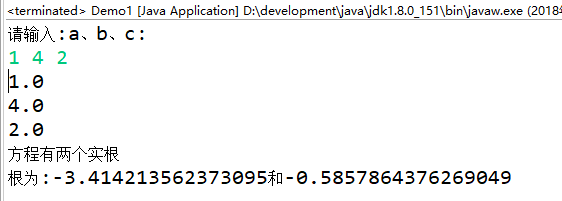代码分析：  链接：https://pan.baidu.com/s/1rv97mmoy2YzNqZN7NuEJYg 密码：lq72
展开全文Java
• 求一元二次方程vb.net源代码 给出方程a,b,c三个系数，可方便出x1、x2两个解。
• 使用c++，只需知道各项系数，利用MFC建立对话框实现求一元一次、二次、三次方程
• 只含有一个未知数（一元），并且未知数项的最高次数是2（二次）的整式方程叫做一元二次方程。vb进行一元二次方程求根程序
• 一元二次方程求根计算器，可以正常显示化简后的分式、根式结果。
• #include<stdio.h> #include<math.h> int main() { double a,b,c,disc,x1,x2,p,q;//定义各变量为double型 ...//输入方程系数 disc=b*b-4*a*c;//b方减去4ac p=-b/(2.0*a); q=sqrt(...
#include<stdio.h> #include<math.h> int main() { double a,b,c,disc,x1,x2,p,q;//定义各变量为double型 scanf("%lf%lf%lf",&a,&b,&c);//输入方程系数 disc=b*b-4*a*c;//求b方减去4ac p=-b/(2.0*a); q=sqrt(disc)/(2.0*a); x1=p+q; x2=p-q; printf("x1=%7.2f\nx2=%7.2f\n",x1,x2);//输出函数根 return 0; }
展开全文• 7-1 求一元二次方程 （20 分） #include “stdafx.h” #include “math.h” int _tmain(int argc, _TCHAR* argv[]) { double a,b,c; scanf("%lf %lf %lf",&amp;a,&amp;b,&amp;c); if(a!...
7-1 求一元二次方程的根 （20 分）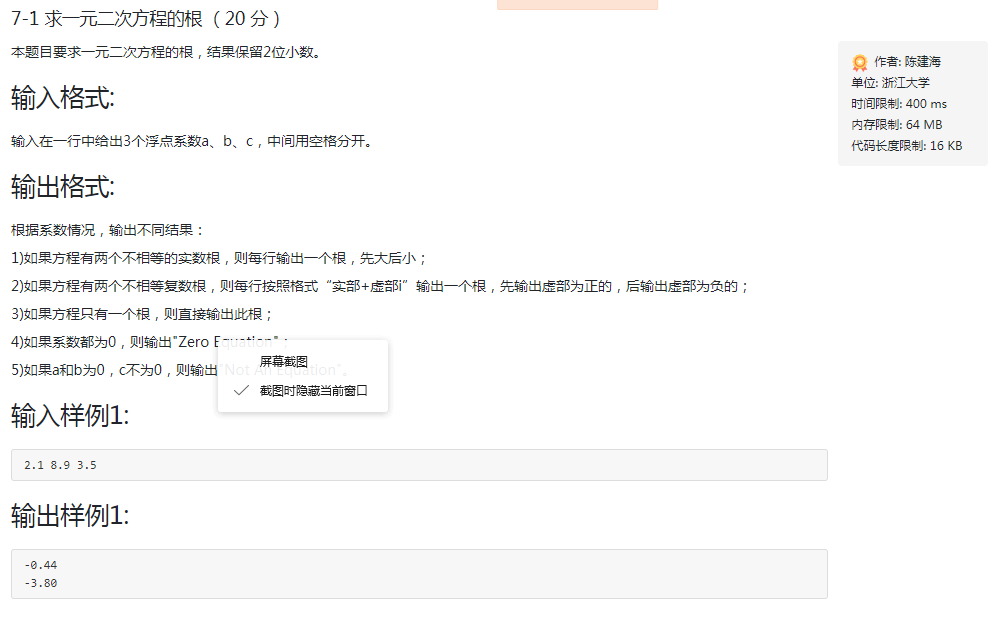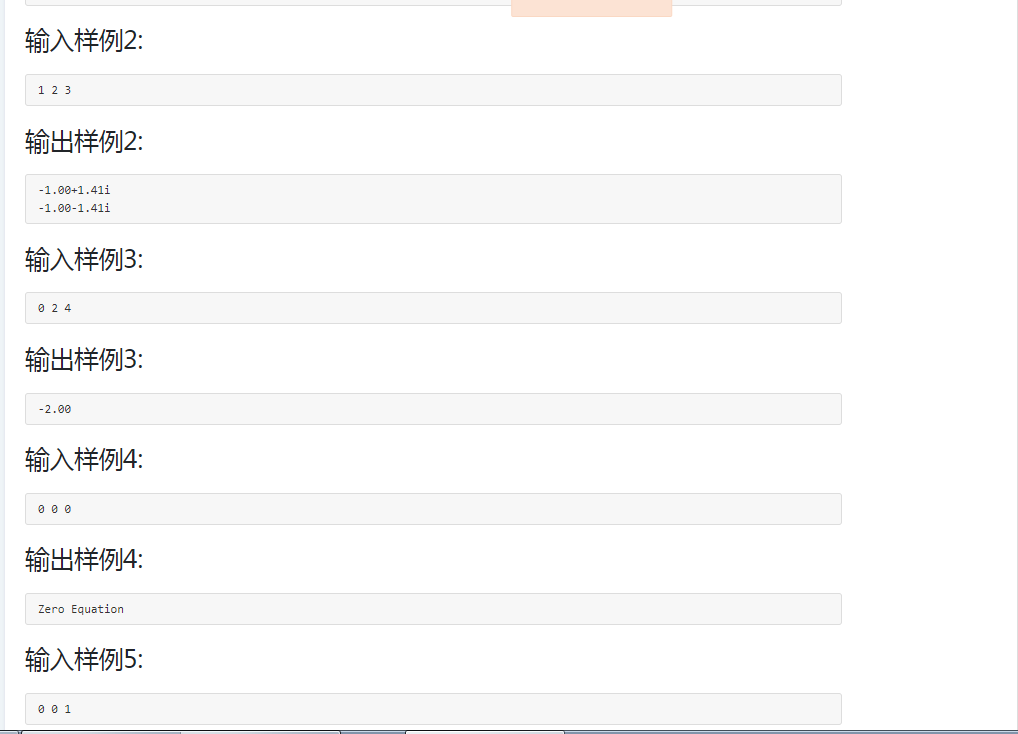#include “stdafx.h”
#include “math.h” int _tmain(int argc, _TCHAR* argv[]) { double a,b,c; scanf("%lf %lf %lf",&a,&b,&c); if(a!=0) { double delta=bb-4ac; if(delta>0) { double x1,x2; x1=(-b+sqrt(delta))/(2a); x2=(-b-sqrt(delta))/(2a); printf("%.2f\n",x1); printf("%.2f\n",x2); } else if(delta<0) { if(b!=0) { double real=-b1.0/(2a); double imag=sqrt(-delta)1.0/(2a); printf("%.2f%+.2fi\n",real,imag); printf("%.2f%+.2fi\n",real,-imag); } else { double imag=sqrt(-delta)1.0/(2a); printf("%.2f%+.2fi\n",0.0,imag); printf("%.2f%+.2fi\n",0.0,-imag); } } else { printf("%.2f\n",-b1.0/(2a)); } } else { if(b!=0) { printf("%.2f\n",-c1.0/b); } else { if(0==c) { printf(“Zero Equation\n”); } else { printf(“Not An Equation\n”); } } } return 0; }
展开全文• 一元二次方程根，java,servlet,利用bean类bao装数据
• 问题 E: 例题3-5 求一元二次方程 时间限制:1 Sec内存限制:128 MB 题目描述 求一元二次方程ax2+bx+c=0的，三个系数a, b, c由键盘输入，且a不能为0，且保证b2-4ac>0。 程序中所涉及的变量均为double类型...C++ codeup
• C++计算并输出一元二次方程 二次方程 ax2+bx+c = 0 (其中a≠0），a 是二次项系数，bx 叫作一次项，b是一次项系数；c叫作常数项。 x 的值为： 的判别式 示例 #include <iostream> #include <cmath&...
• ## c语言求一元二次方程的根

万次阅读 多人点赞 2016-11-15 20:42:08
对于一个一元二次方程进行如下分析：  ax^2+bx+c=0 分析： 1. 当a = 0：  结论：不是一个一元二次方程； 2. 当a！=0：  结论：（1）当b^2-4ac=0,方程有两个相等的实，x1=x2=-b/2a；  （2）当b^2-4.....c语言
• 本题目要求读入3个一元二次方程的系数a,b,c,出该方程的（也可能有复数）。 解题提示：Python的 math模块 中 sqrt 函数负数平方根会报错， 因为math 模块中 sqrt 函数只能进行浮点数的运算。我们知道负数的...
• 利用公式x1 = (-b + sqrt(bb-4ac))/(2a), x2 = (-b - sqrt(bb-4ac))/(2a)求一元二次方程ax2+ bx + c =0的，其中a不等于0。 输入： 输入一行，包含三个浮点数a, b, c（它们之间以一个空格分开），分别表示方程ax2 +...python
• 本文使用Python实现一元二次方程求根公式，主要演示运算符和几个内置函数的用法，封面图片与本文内容无关。def root(a, b, c, highmiddle=True): #首...python 人工智能 机器学习 js tapestry
• 主要介绍了Python实现求解一元二次方程的方法,涉及Python基于math包进行数值运算相关操作技巧,需要的朋友可以参考下
• print("根据a*x^2+b*x+c=0,在a≠0的情况下，有两个，x1=（-b+√（b^2-4ac）/2a和x2=(-b-√(b^2-4ac))/2a）\n2x^2+3x+1=0的是多少") while 1: try: a,b,c=input("输入a、b、c对应的三个数，用空格隔开,a≠0：")...
• 002:求一元二次方程 查看 提交 统计 提问 总时间限制: 1000ms 内存限制: 65536kB 描述 利用公式x1 = (-b + sqrt(b*b-4*a*c))/(2*a), x2 = (-b - sqrt(b*b-4*a*c))/(2*a)求一元二次方程ax2+ bx + c =0的，其中...算法 c语言
• 求一元二次方程 ax2+bx+c=0ax2+bx+c=0ax^2+bx+c=0 的，分情况讨论，结果保留2位小数。 02. 一元二次方程ax2+bx+c=0ax2+bx+c=0ax^2+bx+c=0 的 由代数基本定理，一元二次方程有且仅有两个（重按重数计算...java
• 用Java编写程序，求一元二次方程ax²+bx+c=0的（系数在程序中给定），并输出。java
• 软件测试中涉及到的一元二次求根（实和复根）的代码，可以根据代码设计测试用例，使之达到分支覆盖准则。
• 利用C语言判断一元二次方程的情况，并且一元二次方程
• 该资源可在EXCEL中实现智能化求解一元二次方程，简单易懂，直接按照步骤输入系数即可算出结果，可以直接用，解放了双手，比代码实现还简单！...